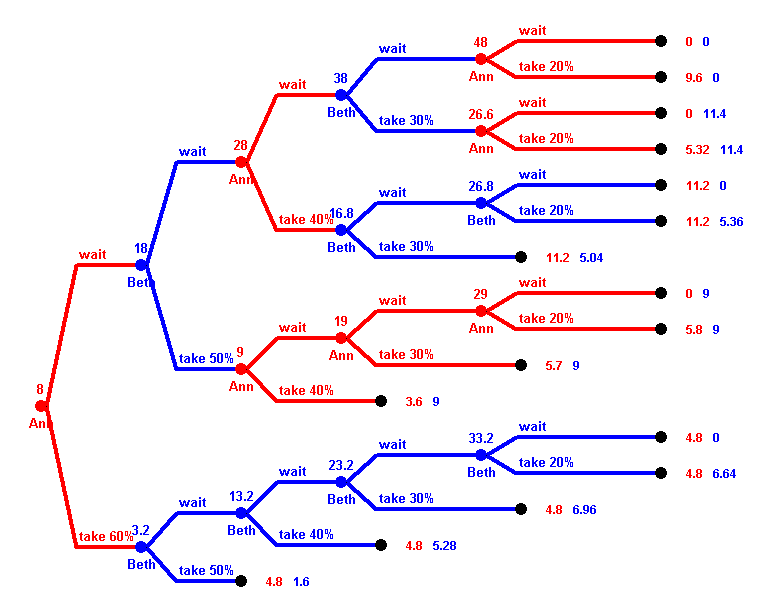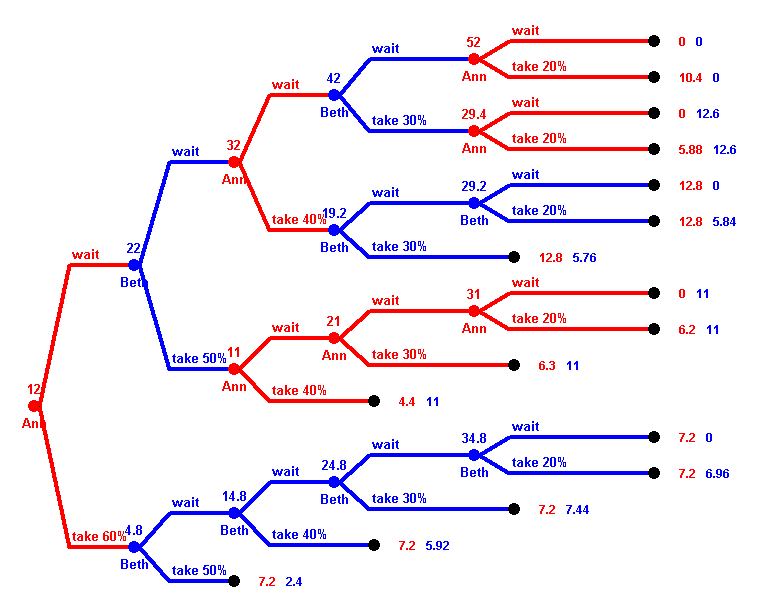# Solutions for Test 2

### First Version

Question 1: Two players, Ann and Beth, play the following game. At the beginning 8 Dollars lie on the desk. There are 5 rounds, where, starting with Ann, the players that have not yet "finished" alternately move. A player who is to move can either take part of the money on the desk, or wait. A player can only take money once---a player who takes some money has "finished" and cannot move anymore. If one player has already finished, the other one moves every round. In the first round, the player who is to move can take 60% of the money, in the second round 50%, in the third round 40%, and so on. But in rounds where no money is taken, where the player waits, the money on the desk increases by \$10.

So, on the one hand, the money increases if the players wait, on the other hand the percentage they are allowed to take decreases over time, and moreover the money can decreases when the other player takes some. So the trick is to find the right time to take money.

The game tree is shown above. At every vertex the amount of money on the desk at that time is written above the vertex, and the name of the player to move below. At the terminal vertices, the payoffs for Ann and beth are given.

Use backwards induction to answer the following questions:

1. (1 point) What is Ann's first move, when playing optimally?
2. (1 point) What is Beth's move in round 2 when she plays optimally? Does it depend on Ann's previous move? If yes, how?
3. (1 point) How much money does Ann and Beth get when both play optimally?
4. (1 point) If Ann plays optimally but Beth not necessarily, how much money can Ann win? Is there some minimum she still can guarantee?From this, the answers are obvious:
1. Ann will wait---the money is simply too low, even with a 60% grab.
2. Beth will take her 50% in this round, provided Ann waited. But if Ann took her 60% in the first round, then Beth would wait, let the money increase, and take it only later.
3. Ann gets 5.8 and Beth 9. It is unfair to Ann.
4. She will win at least 5.8. It could be more, if Beth plays not optimal.

Question 2:
a) (1 point) What is the probability of drawing a king from a deck of 52 cards?
b) (1 point) What is the probability of drawing a red card or a king from a deck of 52 cards?

a) There are 4 kings, each card is equally likely, therefore the probability is 4/52.
b) There are 26 red cards and 4 kings, but two of the kings are also red, therefore totally 26+4-2=28 cards are red or king. Therefore the probability is 28/52.

Question 3:
(1 point) An experiment has three equally likely outcomes. The payoffs for them are \$2,\$3, and -\$1. What is the expected payoff of the experiment?

2/3 + 3/3 + (-1)/3 = 4/3.

Question 4:
(1 points) You have four coins in your pocket: one Euro coins, two 50c, and one 10c. You choose on randomly. What is the expected value?

(1 points) In the same situation as above, what is the expected value if you pick two coins randomly?

a) It is 1/4 · 100 + 2/4 · 50 + 1/4 · 10 = 210/4 = 52.5 cents.
b) Assume one 50c coin is marked. There are six equally likely outcomes. You could get the Euro and the marked 50c (worth 1.50), or the Euro and the unmarked 50c (worth 1.50), or the Euro and the 10c (worth 1.10), or the marked 50c and the unmarked 50c (worth 1.00), or the marked 50c and the 10c (worth 0.60), or the unmarked 50c and the 10c (worth 0.60). The expected value is (1.50+1.50+1.10+1.00+0.60+0.60)/6 = 6.30/6 = 1.05 Euro. It is not a coincidence that this is twice the result in (a). Expected value is additive!

### Second Version

Question 1: Two players, Ann and Beth, play the following game. At the beginning 12 Dollars lie on the desk. There are 5 rounds, where, starting with Ann, the players that have not yet "finished" alternately move. A player who is to move can either take part of the money on the desk, or wait. A player can only take money once---a player who takes some money has "finished" and cannot move anymore. If one player has already finished, the other one moves every round. In the first round, the player who is to move can take 60% of the money, in the second round 50%, in the third round 40%, and so on. But in rounds where no money is taken, where the player waits, the money on the desk increases by \$10.

So, on the one hand, the money increases if the players wait, on the other hand the percentage they are allowed to take decreases over time, and moreover the money can decreases when the other player takes some. So the trick is to find the right time to take money.

The game tree is shown above. At every vertex the amount of money on the desk at that time is written above the vertex, and the name of the player to move below. At the terminal vertices, the payoffs for Ann and beth are given.

Use backwards induction to answer the following questions:

1. (1 point) What is Ann's first move, when playing optimally?
2. (1 point) What is Beth's move in round 2 when she plays optimally? Does it depend on Ann's previous move? If yes, how?
3. (1 point) How much money does Ann and Beth get when both play optimally?
4. (1 point) If Ann plays optimally but Beth not necessarily, how much money can Ann win? Is there some minimum she still can guarantee?From this, the answers are obvious:
1. Ann will take the money immediately---the amount is high enough here.
2. Beth will take her 50% in this round, provided Ann waited. But if Ann took her 60% in the first round, then Beth would wait, let the money increase, and take it only later.
3. Ann gets 7.2 and Beth 7.44. It is almost fair.
4. She will win at least 7.2. It could be more, if Beth plays not optimal.

Question 2:
a) (1 point) What is the probability of drawing a spade from a deck of 52 cards?
b) (1 point) What is the probability of drawing a spade or a queen from a deck of 52 cards?

a) There are 13 spades, each card is equally likely, therefore the probability is 13/52.
b) There are 13 spades and 4 queens, but one queen is also a spade, therefore totally 13+4-1=16 cards are spade or queen. Therefore the probability is 16/52.

Question 3:
(1 point) An experiment has four equally likely outcomes. The payoffs for them are \$1,\$3, \$5, and -\$2. What is the expected payoff of the experiment?

1/4 + 3/4 + 5/4 + (-2)/4 = 7/4.

Question 4:
(1 points) You have four coins in your pocket: one Euro coins, one 50c, and two 20c. You choose on randomly. What is the expected value?

(1 points) In the same situation as above, what is the expected value if you pick two coins randomly?

a) It is 1/4 · 100 + 1/4 · 50 + 2/4 · 20 = 190/4 = 47.5 cents.
b) Assume one 20c coin is marked. There are six equally likely outcomes. You could get the Euro and the 50c (worth 1.50), or the Euro and the marked 20c (worth 1.20), or the Euro and the unmaked 20c (worth 1.20), or the 50c and the marked 20c (worth 0.70), or the 50c and the unmarked 20c (worth 0.70), or the both 20c coins (worth 0.40). The expected value is (1.50+1.20+1.20+0.70+0.70+0.40)/6 = 5.70/6 = 95 cents. It is not a coincidence that this is twice the result in (a). Expected value is additive!